# Lecture 1 SIC Architecture COP 3402 Fall 2007

• Slides: 18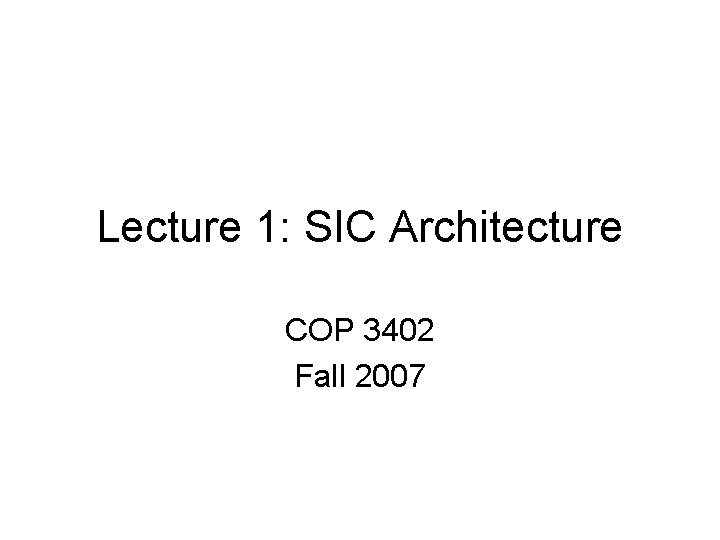Lecture 1: SIC Architecture COP 3402 Fall 2007Simplified Instructional Computer (SIC) • Memory: – 8 -bit bytes – 3 consecutive bytes form a word (24 -bits) – Addresses are byte addresses – Words are addressed by location of their lowest numbered byte – Memory size = 32, 768 (2^15) bytes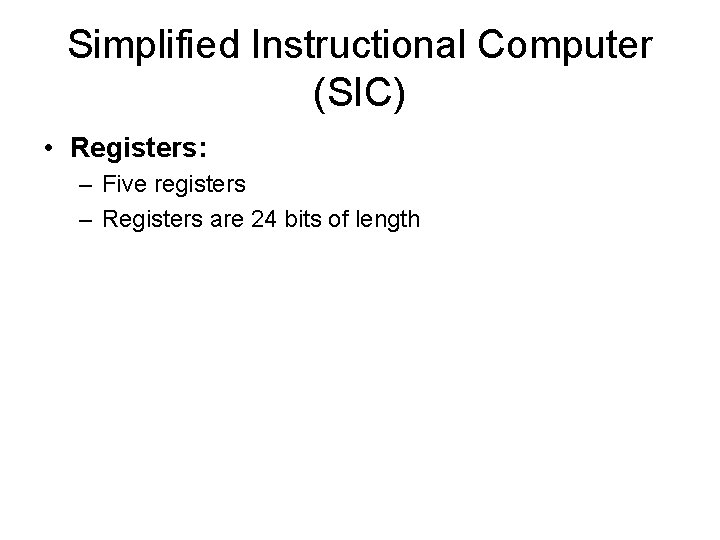Simplified Instructional Computer (SIC) • Registers: – Five registers – Registers are 24 bits of length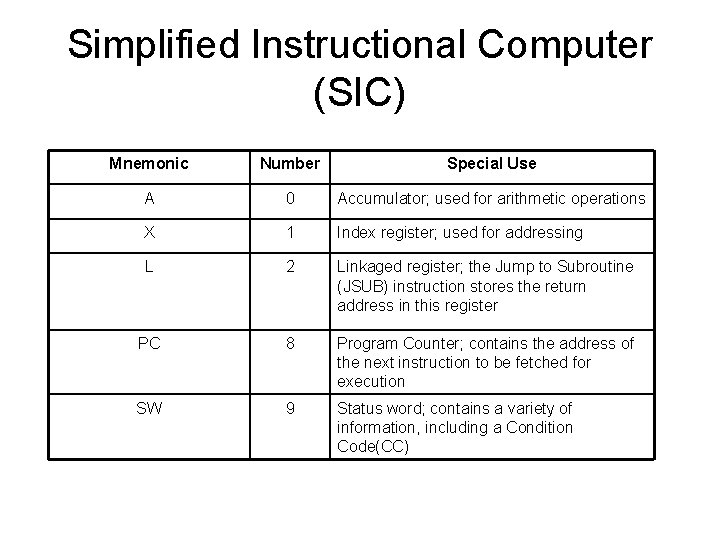Simplified Instructional Computer (SIC) Mnemonic Number Special Use A 0 Accumulator; used for arithmetic operations X 1 Index register; used for addressing L 2 Linkaged register; the Jump to Subroutine (JSUB) instruction stores the return address in this register PC 8 Program Counter; contains the address of the next instruction to be fetched for execution SW 9 Status word; contains a variety of information, including a Condition Code(CC)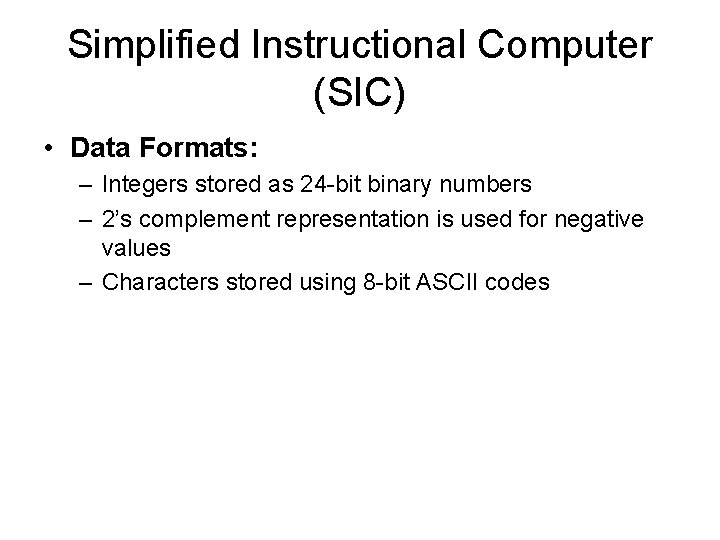Simplified Instructional Computer (SIC) • Data Formats: – Integers stored as 24 -bit binary numbers – 2’s complement representation is used for negative values – Characters stored using 8 -bit ASCII codes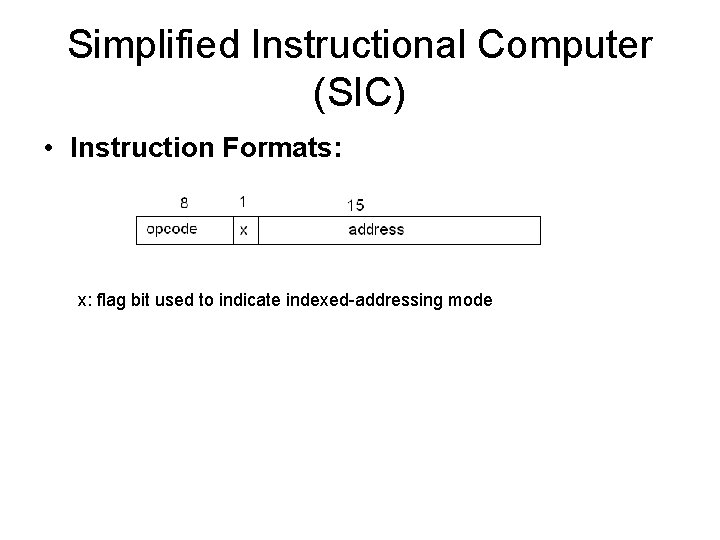Simplified Instructional Computer (SIC) • Instruction Formats: x: flag bit used to indicate indexed-addressing mode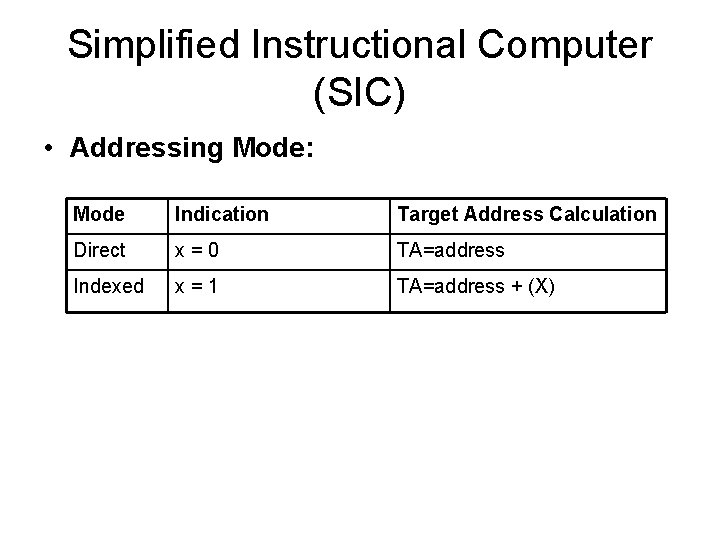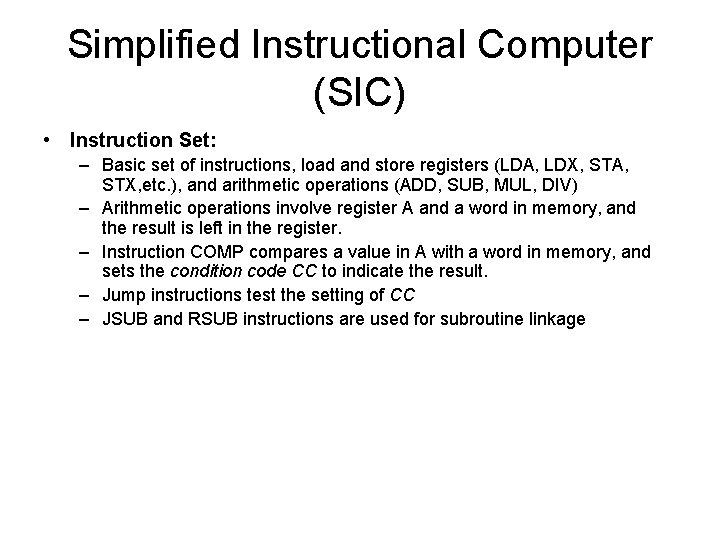Simplified Instructional Computer (SIC) • Instruction Set: – Basic set of instructions, load and store registers (LDA, LDX, STA, STX, etc. ), and arithmetic operations (ADD, SUB, MUL, DIV) – Arithmetic operations involve register A and a word in memory, and the result is left in the register. – Instruction COMP compares a value in A with a word in memory, and sets the condition code CC to indicate the result. – Jump instructions test the setting of CC – JSUB and RSUB instructions are used for subroutine linkage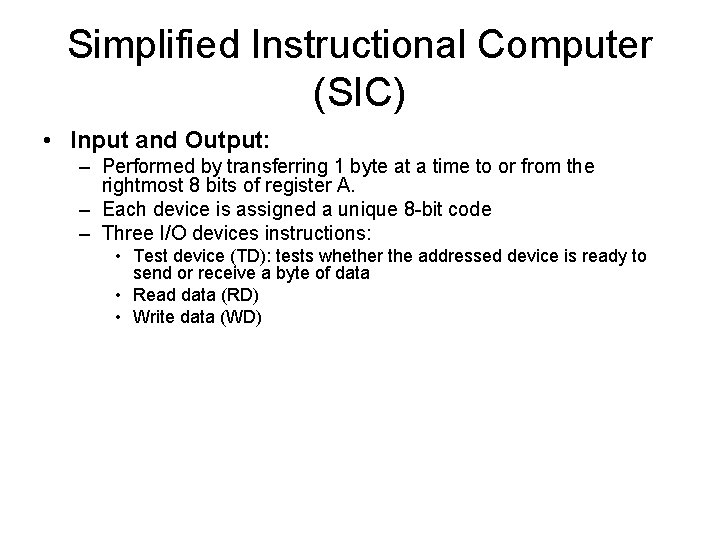Simplified Instructional Computer (SIC) • Input and Output: – Performed by transferring 1 byte at a time to or from the rightmost 8 bits of register A. – Each device is assigned a unique 8 -bit code – Three I/O devices instructions: • Test device (TD): tests whether the addressed device is ready to send or receive a byte of data • Read data (RD) • Write data (WD)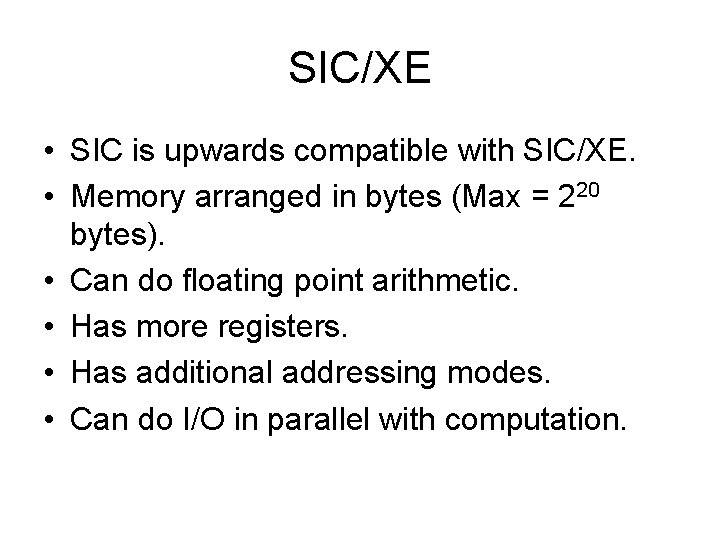SIC/XE • SIC is upwards compatible with SIC/XE. • Memory arranged in bytes (Max = 220 bytes). • Can do floating point arithmetic. • Has more registers. • Has additional addressing modes. • Can do I/O in parallel with computation.Registers Mnemonic Register Comment 3 B Base Register (for addressing) 4 S General Purpose Register 5 T General Purpose Register 6 F Floating point Accumalator (48 -bits)Data Formats • SIC/XE supports integers and characters in the same manner as SIC. • Introduces new 48 -bit floating point type. • 1 -bit sign bit • 11 -bit exponent • 36 -bit fractionFloating point format in SIC/XE • 0 <= fraction <=1 • Exponent is unsigned. Range = (0 – 2047) • Subtract 1024 from exponent to get correct value. • Value of any float will be. Fraction * (2 exponent-1024) • The sign of the number will be determined by the sign bit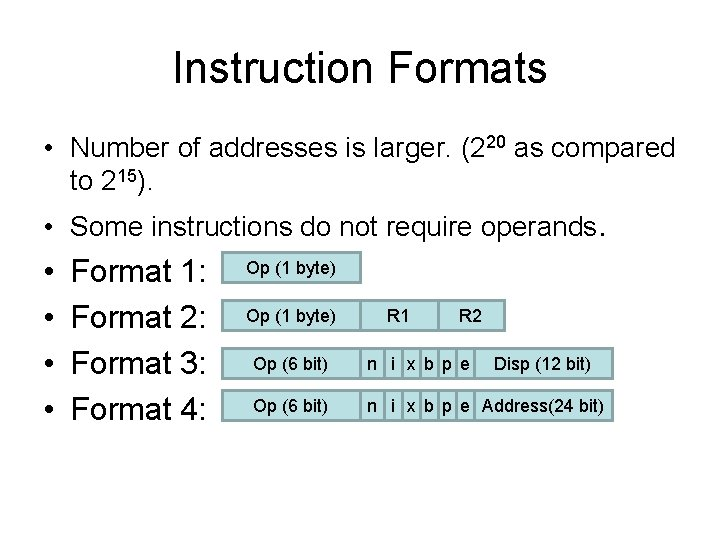Instruction Formats • Number of addresses is larger. (220 as compared to 215). • Some instructions do not require operands. • • Format 1: Format 2: Format 3: Format 4: Op (1 byte) R 1 R 2 Op (6 bit) n i x b p e Disp (12 bit) Op (6 bit) n i x b p e Address(24 bit)Formats (cont’d) • n=1, i=0: The word at the target address is fetched. • n=0, i=1: The address is used as the operand. • n=0, i=0: value at address is taken as operand. (backward compatibility with SIC when used in Format 3) • x=0, 1: enables/disables Indexed mode (as in SIC).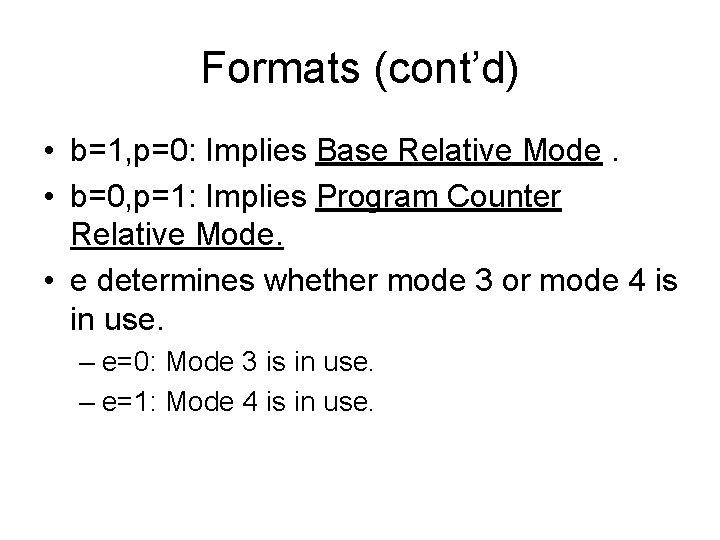Formats (cont’d) • b=1, p=0: Implies Base Relative Mode. • b=0, p=1: Implies Program Counter Relative Mode. • e determines whether mode 3 or mode 4 is in use. – e=0: Mode 3 is in use. – e=1: Mode 4 is in use.Addressing Modes • Base Relative: b=1, p=0 – Target Address = B + disp • Program Counter Relative: b=0, p=1 – Target Address = PC+disp • If b=p=0, then the address/disp field is taken as the address. • Indexed addressing may be used with both these modes (x=0, 1).Instructions • Instructions to Load/Store new registers – LDB, STB, … • Floating point Arithmetic – ADDF, SUBF, MULF, DIVF • Register Instructions – ADDR, SUBR, MULR, DIVR, RMO • I/O instructions – TIO, SIO, HIO